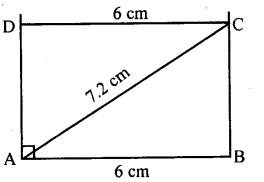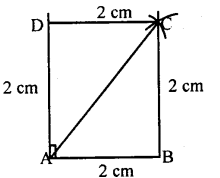# KSEEB Solutions for Class 8 Maths Chapter 15 Quadrilaterals Ex 15.5

Students can Download Maths Chapter 15 Quadrilaterals Ex 15.5 Questions and Answers, Notes Pdf, KSEEB Solutions for Class 8 Maths helps you to revise the complete Karnataka State Board Syllabus and score more marks in your examinations.

## Karnataka Board Class 8 Maths Chapter 15 Quadrilaterals Ex 15.5

Question 1.
Construct rectangle ABCD with the following data.
(a) AB = 4 cm, BC = 6 cm.
Opposite sides of a rectangle are equal as each angle is a right angle.
∴ AB = CD = 4 cm.
Steps:1. Draw a line segment AB = 6 cm.
2. Construct a perpendicular at B.
3. With a 7 cm radius at A as center draw an arc on the perpendicular. Let the point be C.
4. With BC as radius draw an arc from A.
5. With AB as radius cut the arc from A.

(b) AB = 6 cm AC = 7.2 cm
Steps:1. Draw a line segment AB = 6 cm.
2. Draw perpendicular at B.
3. With a 4 cm radius cut the line at A.
4. With AD = 6 cm draw an arc.
5. With CD – 4 cm cut the arc.

Question 2.
Construct square ABCD
(a) Which has side length 2 cm.
(b) Which has diagonal 6 cm.
(a). Steps1. Draw a line segment AB = 2 cm.
2. At A, construct a perpendicular
3. With A as center cut the perpendicular
4. With a 2 cm radius from B and D draw the arc.
5. Join DC and BC.

(b) Steps:1. Draw a line segment AC = 6 cm.
2. Construct a perpendicular bisector to AC intersecting at O.
3. With O as the center and 3cm radius draw the line on both sides cutting at B and D.
4. Join AB, AD, DC & BC.
error: Content is protected !!